# 对不起，我错了，这题不简单！

justtouch

0收藏

hello，大家好呀，我是小楼。``````const (
UNINITIALIZED = iota
IDLE
PREPARE
RUNNING
)``````

``````type statistic struct {
writes uint64
}

func (s *statistic) NewEmptyBucket() interface{} {
return statistic{
writes: 0,
}
}

func (s *statistic) ResetBucketTo(bucket *base.BucketWrap, startTime uint64) *base.BucketWrap {
atomic.StoreUint64(&bucket.BucketStart, startTime)
bucket.Value.Store(statistic{
writes: 0,
})
return bucket
}``````

``````func currentCounter(now uint64) (*statistic, error) {
if statistics == nil {
return nil, fmt.Errorf("statistics is nil")
}

bk, err := statistics.CurrentBucketOfTime(now, bucketGenerator)
if err != nil {
return nil, err
}
if bk == nil {
return nil, fmt.Errorf("current bucket is nil")
}

if v == nil {
return nil, fmt.Errorf("current bucket value is nil")
}
counter, ok := v.(*statistic)
if !ok {
return nil, fmt.Errorf("bucket fail to do type assert, expect: *statistic, in fact: %s", reflect.TypeOf(v).Name())
}

return counter, nil
}``````

``````func currentQps(now uint64) (uint64, uint64) {
if statistics == nil {
return 0, 0
}

list := statistics.ValuesConditional(now, func(ws uint64) bool {
return ws <= now && now < ws+uint64(bucketLengthInMs)
})

for _, w := range list {
if w == nil {
continue
}

if v == nil {
continue
}

s, ok := v.(*statistic)
if !ok {
continue
}

cnt++
writes += s.writes
}

if cnt < 1 {
return 0, 0
}

return reads / cnt, writes / cnt
}``````

``````func check() {
now := CurrentTimeMillsWithTicker(true)
if now-lastCheck < checkInterval {
return
}

lastCheck = now
qps, tps := currentQps(now)
if state == IDLE && qps > hitsUpperBoundary {
logging.Warn("[time_ticker check] switches to PREPARE for better performance", "reads", qps, "writes", tps)
state = PREPARE
} else if state == RUNNING && qps < hitsLowerBoundary {
logging.Warn("[time_ticker check] switches to IDLE due to not enough load", "reads", qps, "writes", tps)
state = IDLE
}
}``````

``````func StartTimeTicker() {
var err error
statistics, err = base.NewLeapArray(sampleCount, intervalInMs, bucketGenerator)
if err != nil {
logging.Warn("[time_ticker StartTimeTicker] new leap array failed", "error", err.Error())
}

atomic.StoreUint64(&nowInMs, uint64(time.Now().UnixNano())/unixTimeUnitOffset)
state = IDLE
go func() {
for {
check()
if state == RUNNING {
now := uint64(time.Now().UnixNano()) / unixTimeUnitOffset
atomic.StoreUint64(&nowInMs, now)
counter, err := currentCounter(now)
if err != nil && counter != nil {
}
time.Sleep(time.Millisecond)
continue
}
if state == IDLE {
time.Sleep(300 * time.Millisecond)
continue
}
if state == PREPARE {
now := uint64(time.Now().UnixNano()) / unixTimeUnitOffset
atomic.StoreUint64(&nowInMs, now)
state = RUNNING
continue
}
}
}()
}``````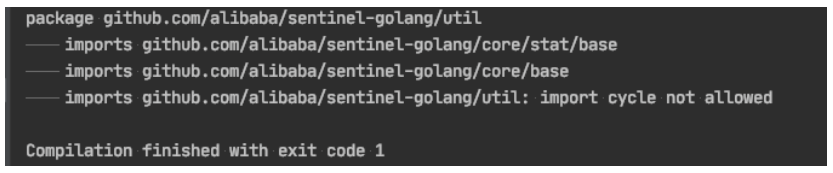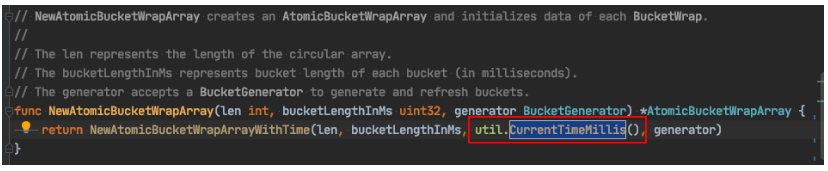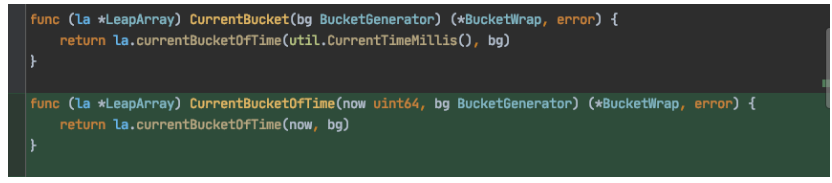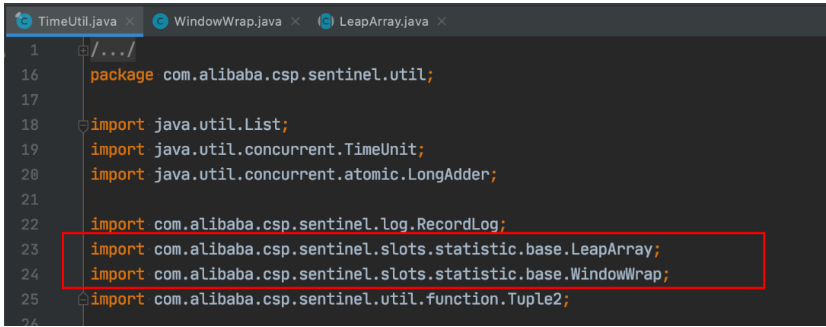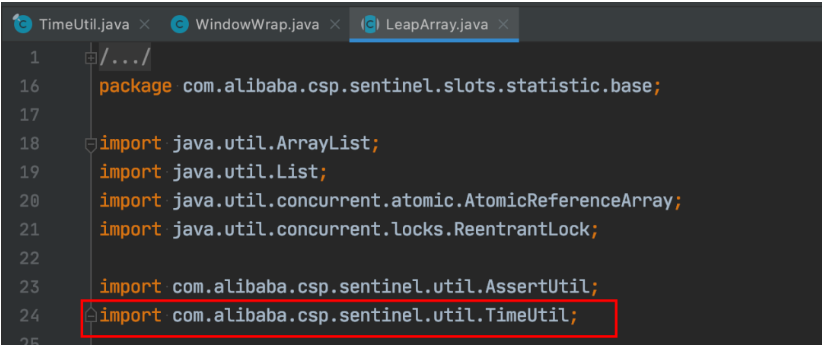Java也出现了循环依赖，但它没事！

``````package org.newboo.pk1;

import org.newboo.pk2.Test2;

public class Test1 {
public static int code() {
return Test2.code();
}

public static void main(String[] args) {
System.out.println(code());
}
}``````

``````Exception in thread "main" java.lang.StackOverflowError
at org.newboo.pk1.Test1.code(Test1.java:7)
at org.newboo.pk2.Test2.code(Test2.java:7)
...``````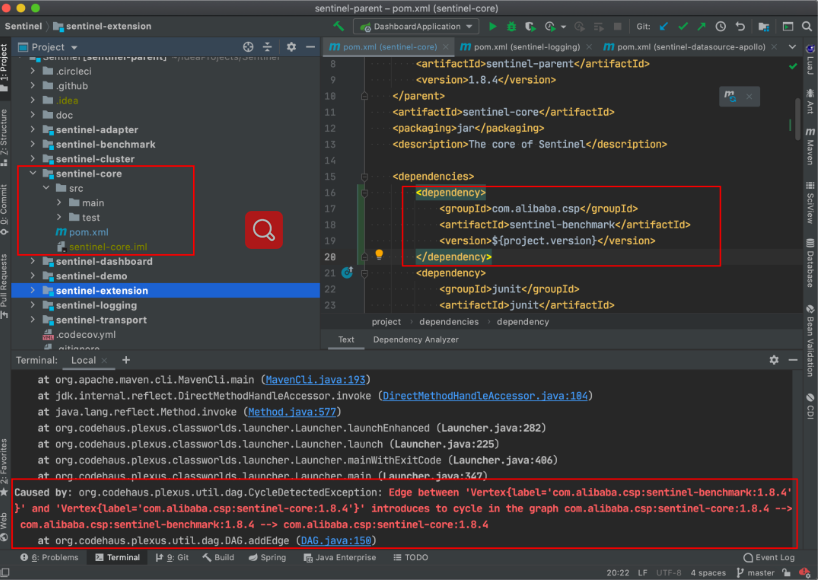Java中强制禁止的只有maven，语言层面、框架层面基本都没有赶尽杀绝，但Go却在语言层面强制不让使用。

``````---- util
---- ---- common
---- base
---- ---- common``````

``````// Returns the current Unix timestamp in milliseconds.
func CurrentTimeMillis() uint64 {
return CurrentClock().CurrentTimeMillis()
}``````

``````type Clock interface {
Now() time.Time
Sleep(d time.Duration)
CurrentTimeMillis() uint64
CurrentTimeNano() uint64
}``````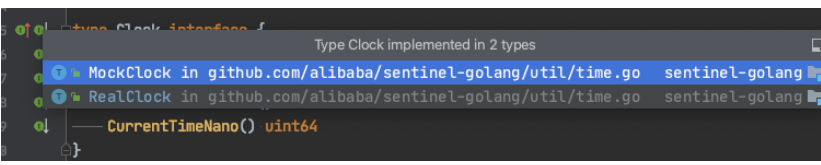``````func init() {
realClock := NewRealClock()
currentClock = new(atomic.Value)
SetClock(realClock)

realTickerCreator := NewRealTickerCreator()
currentTickerCreator = new(atomic.Value)
SetTickerCreator(realTickerCreator)
}``````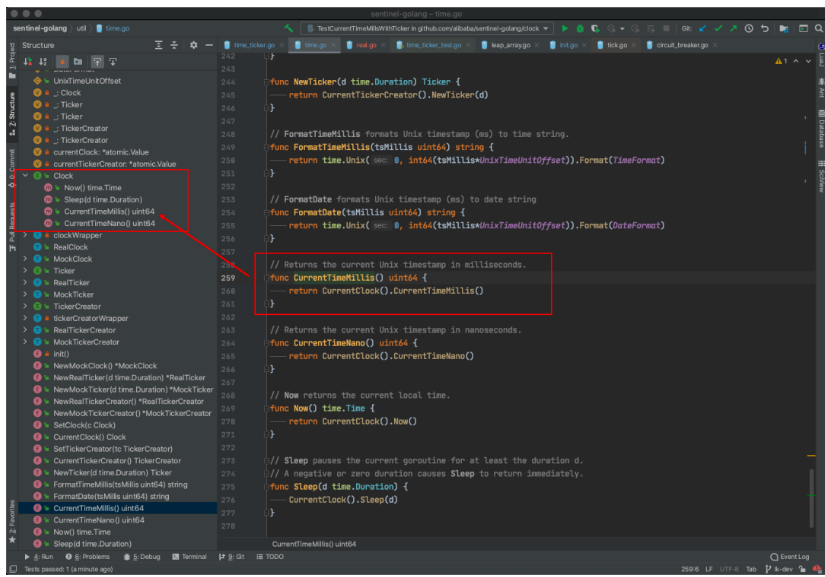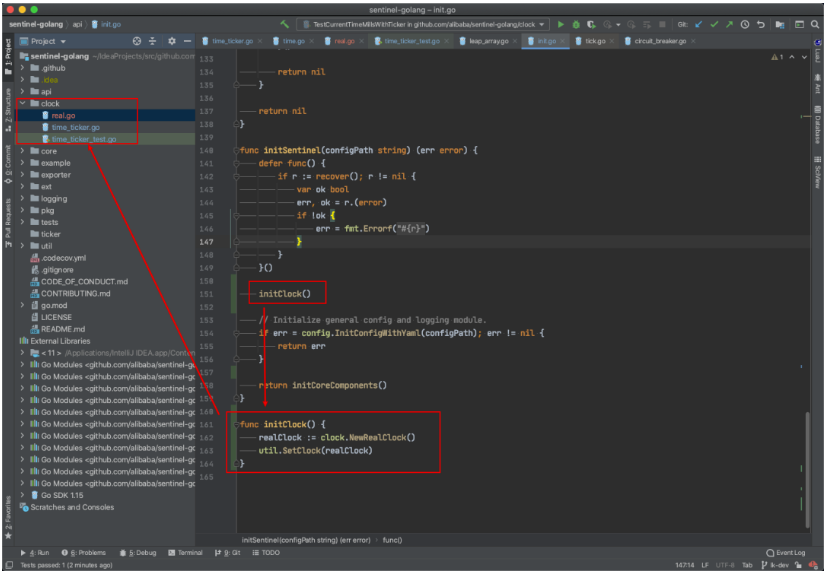《惨，给Go提的代码被批麻了》帖子
视频
声望
粉丝
相关问题
社区精华内容